## Saturday, May 28, 2016

### Ruby operators: equality, comparison, pattern matching and ordering

An operator is a character or a small set of characters that represent an action which is applied to one or more operands. Ruby provides many different kinds of operators; this post covers equality, comparison, pattern matching and ordering operators, all of which are implemented as methods. When one of those operators is reached within the code, it calls the corresponding method. When we type 2+3, we are actually calling the + method of the Integer class and providing 3 as an argument. We can rewrite it as 2.+(3) and get the same result.

Operator overloading means changing the behavior of an operator by overriding its corresponding method. That is, however, outside the scope of this post.

## Equality operators: == and !=

The == operator, also known as equality or double equal, will return true if both objects are equal and false if they are not.

``````
"koan" == "koan" # Output: => true
``````

The != operator, AKA inequality or bang-tilde, is the opposite of ==. It will return true if both objects are not equal and false if they are equal.

``````
"koan" != "discursive thought" # Output: => true
``````

Note that two arrays with the same elements in a different order are not equal, uppercase and lowercase versions of the same letter are not equal and so on.

When comparing numbers of different types (e.g., integer and float), if their numeric value is the same, == will return true.

``````
2 == 2.0 # Output: => true
``````

## Additional methods for testing equality

### equal?

Unlike the == operator which tests if both operands are equal, the equal method checks if the two operands refer to the same object. This is the strictest form of equality in Ruby.

Example:
``````
a = "zen"
b = "zen"

a.object_id  # Output: => 20139460
b.object_id  # Output :=> 19972120

a.equal? b  # Output: => false
``````

In the example above, we have two strings with the same value. However, they are two distinct objects, with different object IDs. Hence, the equal? method will return false.

Let's try again, only this time b will be a reference to a. Notice that the object ID is the same for both variables, as they point to the same object.

``````
a = "zen"
b = a

a.object_id  # Output: => 18637360
b.object_id  # Output: => 18637360

a.equal? b  # Output: => true
``````

### eql?

In the Hash class, the eql? method it is used to test keys for equality. Some background is required to explain this. In the general context of computing, a hash function takes a string (or a file) of any size and generates a string or integer of fixed size called hashcode, commonly referred to as only hash. Some commonly used hashcode types are MD5, SHA-1, and CRC. They are used in encryption algorithms, database indexing, file integrity checking, etc. Some programming languages, such as Ruby, provide a collection type called hash table. Hash tables are dictionary-like collections which store data in pairs, consisting of unique keys and their corresponding values. Under the hood, those keys are stored as hashcodes. Hash tables are commonly referred to as just hashes. Notice how the word hash can refer to a hashcode or to a hash table. In the context of Ruby programming, the word hash almost always refers to the dictionary-like collection.

Ruby provides a built-in method called hash for generating hashcodes. In the example below, it takes a string and returns a hashcode. Notice how strings with the same value always have the same hashcode, even though they are distinct objects (with different object IDs).

``````
"meditation".hash  # Output: => 1396080688894079547
"meditation".hash  # Output: => 1396080688894079547
"meditation".hash  # Output: => 1396080688894079547
``````

The hash method is implemented in the Kernel module, included in the Object class, which is the default root of all Ruby objects. Some classes such as Symbol and Integer use the default implementation, others like String and Hash provide their own implementations.

``````
Symbol.instance_method(:hash).owner  # Output: => Kernel
Integer.instance_method(:hash).owner # Output: => Kernel

String.instance_method(:hash).owner  # Output: => String
Hash.instance_method(:hash).owner  # Output: => Hash
``````

In Ruby, when we store something in a hash (collection), the object provided as a key (e.g., string or symbol) is converted into and stored as a hashcode. Later, when retrieving an element from the hash (collection), we provide an object as a key, which is converted into a hashcode and compared to the existing keys. If there is a match, the value of the corresponding item is returned. The comparison is made using the eql? method under the hood.

``````
"zen".eql? "zen"    # Output: => true
# is the same as
"zen".hash == "zen".hash # Output: => true
``````

In most cases, the eql? method behaves similarly to the == method. However, there are a few exceptions. For instance, eql? does not perform implicit type conversion when comparing an integer to a float.

``````
2 == 2.0    # Output: => true
2.eql? 2.0    # Output: => false
2.hash == 2.0.hash  # Output: => false
``````

## Case equality operator: ===

Many of Ruby's built-in classes, such as String, Range, and Regexp, provide their own implementations of the === operator, also known as case-equality, triple equals or threequals. Because it's implemented differently in each class, it will behave differently depending on the type of object it was called on. Generally, it returns true if the object on the right "belongs to" or "is a member of" the object on the left. For instance, it can be used to test if an object is an instance of a class (or one of its subclasses).

``````
String === "zen"  # Output: => true
Range === (1..2)   # Output: => true
Array === [1,2,3]   # Output: => true
Integer === 2   # Output: => true
``````

The same result can be achieved with other methods which are probably best suited for the job, such as is_a? and instance_of?.

### Range Implementation of ===

When the === operator is called on a range object, it returns true if the value on the right falls within the range on the left.

``````
(1..4) === 3  # Output: => true
(1..4) === 2.345 # Output: => true
(1..4) === 6  # Output: => false

("a".."d") === "c" # Output: => true
("a".."d") === "e" # Output: => false
``````

Remember that the === operator invokes the === method of the left-hand object. So (1..4) === 3 is equivalent to (1..4).=== 3. In other words, the class of the left-hand operand will define which implementation of the === method will be called, so the operand positions are not interchangeable.

### Regexp Implementation of ===

Returns true if the string on the right matches the regular expression on the left.
``````
/zen/ === "practice zazen today"  # Output: => true
# is similar to
"practice zazen today"=~ /zen/
``````

The only relevant difference between the two examples above is that, when there is a match, === returns true and =~ returns an integer, which is a truthy value in Ruby. We will get back to this soon.

### Implicit usage of the === operator on case/when statements

This operator is also used under the hood on case/when statements. That is its most common use.

``````
minutes = 15

case minutes
when 10..20
puts "match"
else
puts "no match"
end

# Output: match
``````

In the example above, if Ruby had implicitly used the double equal operator (==), the range 10..20 would not be considered equal to an integer such as 15. They match because the triple equal operator (===) is implicitly used in all case/when statements. The code in the example above is equivalent to:

``````
if (10..20) === minutes
puts "match"
else
puts "no match"
end
``````

## Pattern matching operators: =~ and !~

The =~ (equal-tilde) and !~ (bang-tilde) operators are used to match strings and symbols against regex patterns.

The implementation of the =~ method in the String and Symbol classes expects a regular expression (an instance of the Regexp class) as an argument.

``````
"practice zazen" =~ /zen/   # Output: => 11
"practice zazen" =~ /discursive thought/ # Output: => nil

:zazen =~ /zen/    # Output: => 2
:zazen =~ /discursive thought/  # Output: => nil
``````

The implementation in the Regexp class expects a string or a symbol as an argument.

``````
/zen/ =~ "practice zazen"  # Output: => 11
/zen/ =~ "discursive thought" # Output: => nil
``````

In all implementations, when the string or symbol matches the Regexp pattern, it returns an integer which is the position (index) of the match. If there is no match, it returns nil. Remember that, in Ruby, any integer value is "truthy" and nil is "falsy", so the =~ operator can be used in if statements and ternary operators.

``````
puts "yes" if "zazen" =~ /zen/ # Output: => yes
"zazen" =~ /zen/?"yes":"no" # Output: => yes
``````

Pattern-matching operators are also useful for writing shorter if statements. Example:

``````
if meditation_type == "zazen" || meditation_type == "shikantaza" || meditation_type == "kinhin"
true
end
``````
Can be rewritten as:
``````
if meditation_type =~ /^(zazen|shikantaza|kinhin)\$/
true
end
``````

The !~ operator is the opposite of =~, it returns true when there is no match and false if there is a match.

## Comparison operators

Objects such as numbers and strings, which can be compared (amongst themselves) in terms of being greater or smaller than others, provide the <=> method, also known as the spaceship method. When comparing two objects, <=> returns -1 if the first object is lesser than the second (a < b), 0 in case they are equal (a == b) and 1 when the first object is greater than the second (a > b).

``````
5 <=> 8  # Output:  => -1
5 <=> 5  # Output: => 0
8 <=> 5  # Output: => 1
``````

Most comparable or sortable object classes, such as Integer, Float, Time and String, include a mixin called Comparable, which provides the following comparison operators: < (less than), <= (less than or equal), == (equal), > (greater than), >= (greater than or equal). These methods use the spaceship operator under the hood.

Let's find out which classes include the Comparable mixin:

``````
ObjectSpace.each_object(Class).select { |c| c.included_modules.include? Comparable }
``````
Output:
``````
=> [Complex, Rational, Time, File::Stat, Bignum, Float, Fixnum, Integer, Numeric, Symbol, String, Gem::Version, IRB::Notifier::NoMsgNotifier, IRB::Notifier::LeveledNotifier]
``````

Comparison operators can be used in objects of all the above classes, as in the following examples.

``````
# String
"a" < "b" # Output: => true
"a" > "b" # Output: => false

# Symbol
:a < :b  # Output: => true
:a > :b  # Output: => false

# Fixnum (subclass of Integer)
1 < 2  # Output: => true
2 >= 2  # Output: => true

# Float
1.0 < 2.0 # Output: => true
2.0 >= 2.0 # Output: => true

# Time
Time.local(2016, 5, 28) < Time.local(2016, 5, 29) # Output: => true
``````

When comparing numbers of different classes, comparison operators will implicitly perform simple type conversions.

``````
# Fixnum vs. Float
2 < 3.0  # Output: => true
2.0 > 3  # Output: => false
``````

1.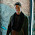Thorough and excellent! Thank you so much!

1.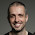I'm glad you enjoyed it. Thank you for reading and providing feedback.

2.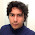Good article. Help me enough, thanks!

1.3.Really cool Bruno!

Other cool thing around comparisons that complements `is_a?` is comparing classes with ancestors using `<` and `>` operators:

irb(main):001:0> Numeric < Float
=> false
irb(main):002:0> 1.class < Numeric
=> true

1.Nice to see you here Jônatas.

Thanks for the tip! I'll be sure to include it in the post I'm writing about classes.

4.Good stuff.

1.Thank you :)

5.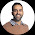Thank you Bruno, informative and concise! Cheers.

1.Thank you for reading. Your feedback means a lot.

6.Your description of "Symbol Implementation of ===" is wrong. It is rather an alias for "==":
:foo.method(:===) == :foo.method(:==) # => true

1.You are right, it was wrong. I have updated the post. Thank you for pointing that out.

7.I have read your blog and I gathered some needful information from your blog. Keep update your blog. Awaiting for your next update.

software testing course in chennai

8.very informative blog and useful article thank you for sharing with us , keep posting
Ruby on Rails Online Training Hyderabad

9.Nice and good article. It is very useful for me to learn and understand easily. Thanks for sharing your valuable information and time. Please keep updating.
Web Design Training

10.Fantastic blog.you set Good stuff.All that the topics were clarified briefly.so immediately know because of mepersonally.phonetracking

11.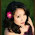This is helpful article. I have read your blog and I gathered some needful information from your blog. Keep update your blog & working hard on that. by the way, you may visit https://proweb365.com/blog to see many value article about web design market in recent years.

12.Sistem bandar dalam permainan ini semakin membuat anda lebih mudah menang dalam bermain karena hanya bertaruh dengan 1 orang pemain. Dikesempatan kali ini
asikqq
dewaqq
sumoqq
interqq
pionpoker
bandar ceme terbaik
hobiqq
paito warna terlengkap
syair sgp